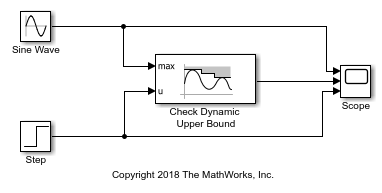# Check Signal Upper Bound with Check Dynamic Upper Bound Block

Using the Check Dynamic Upper Bound block, you can check if an input signal rises above a changing upper bound during a simulation.In this example, the Check Dynamic Upper Bound block compares the value of a tested input signal from a Step block at the u port to a Sine Wave block at the max port. The Check Dynamic Upper Bound block checks if the value of the signal at the max port is greater than the value of the tested input sigal. If it is, the block asserts `true (1)`. Because the Output assertion signal parameter of the Check Dynamic Upper Bound block is selected, the block outputs the assertion value. Run the simulation to observe the model output.At the start of the simulation, the Check Dynamic Upper Bound block outputs `1` because the value of the Sine Wave block is greater than the value of the Step block. At a time of `3.14`, the sine wave dips below `0`, causing the value of the Step block to exceed the sine wave. The Check Dynamic Upper Bound block recognizes this change and outputs `0`.

At a time of `5`, the Step block outputs a value of `0.5`, which is still greater than the sine wave. The Step block value stays greater than the sine wave until the time reaches `6.81`. The Check Dynamic Upper Bound block recognizes this change and the assertion passes. The output stays at `1` until the time reaches `8.90`, where the sine wave goes below the value of the Step block.